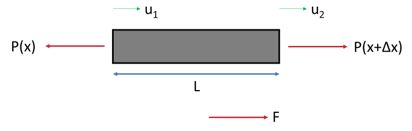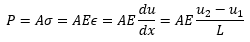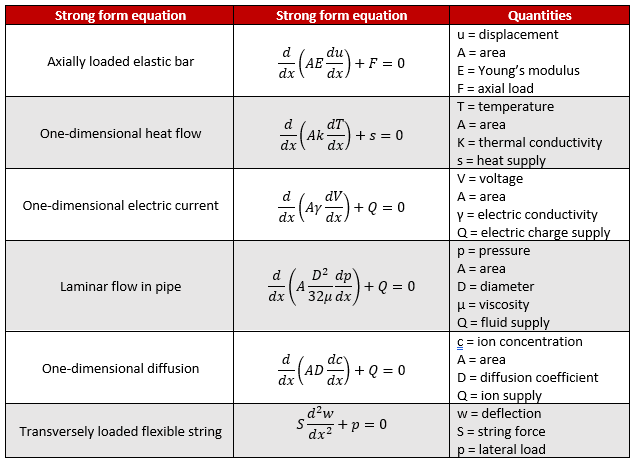top of page
Search

# What Is Finite Element Analysis? And How Does It Really Work?

Mechanical engineers in their studies will learn how to do mechanical analysis on basic shapes, such as beams. However, real life parts are almost never that simple. The shapes are complex and they can be loaded or constrained in various ways. So how can we analyze these parts? The answer is through finite element analysis!

Finite element analysis is an increasingly popular method used to solve problems in static structural analysis, dynamic analysis, vibration analysis, fluid flow, heat transfer, and many more types of physics. Some other applications of finite element analysis include vehicle impact, prosthetic limb testing, and submarine pressure hull testing. But where did it come from and how does it work?

## A Brief History of FEA

While different methods for solving systems of differential equations existed for many centuries, dating back to Euler, some of the first works that utilized these methods to solve problems in mechanics were from Alexander Hrennikoff and Richard Courant in the 1940s. More papers developing the theory were published throughout the 1950s, especially within the aerospace industry. The paper “Stiffness and Deflection Analysis of Complex Structures”, published in 1956 by Turner, Clough, Martin, and Topp is generally considered to be the birth of the current finite element method. The term “finite element” was coined by Ray Clough in 1960. Use of the finite element method did not really take off until computers became sophisticated enough to perform these calculations and with the invention of programs such as NASTRAN in the late 1960s. Today, finite element analysis is very commonly used and can solve many types of complicated physics problems and has also become easier to use. Any designer could easily learn how to set up an analysis using the finite element software out there today. But what is really going on in the background?

## Why Use FEA?

Before we get into the nitty gritty of exactly how FEA works, let's first ask; why would we want to use it? Well, the answer to that is fairly simple - it allows us to test, bend, break, heat, vibrate things without having to build them first. This all sounds cool, but it is actually hugely beneficial to organizations - large and small - to be able to develop confidence in products at the start of the design process, rather than at the end. Imagine having the ability to predict problems or failures in products before they even exist… that is what FEA and other simulation allows us to do.

And from a commercial standpoint, this results in huge savings in both time and money - two of the most valuable things in any design process.

Check out the examples below to get an insight into the types of things that we can simulate using FEA:

### Examples Of FEAStructural analysis of a pipe intersectionStructural analysis of a chairThermal analysis of a pump housingDynamic analysis of pipe whipDynamic analysis of a bird strike

## The Theory Of FEA

OK, now we know why we might want to use it, let's jump into some of the theory of the FEA method. This can be a little bit dry, but it is really useful to have a basic understanding of what the solvers are doing for a number of reasons. Notably, it helps us to better comprehend the capabilities and limitations of the solutions that we're applying, which, in turn, allows us to build better models and more efficient and effective analyses.

The general idea of finite element analysis is to replace a problem P with a discretized Pn. The solution of Pn, Sn, will tend toward the solution of P, S (Courant). It is a numerical method that separates a complex geometry into a mesh. The mesh consists of elements that are connected by nodes. Each element’s displacement can be solved using simpler equations. There are many different types of elements that are suited for different geometries and applications – see this blog for more on that!An example of a part next to its respective mesh. Part courtesy of GrabCAD

### The Stiffness Matrix

Since the elements share nodes, the equations to solve them are linked together. Because of this, they can be expressed in a matrix form:In this equation, K is known as the stiffness matrix, u is the vector of displacements, and F is the vector of forces. This is similar to the way a spring would behave (Hooke’s law: F=kx). The stiffness matrix is the most important part of this equation as it will be used in combination with the known F vector to solve for u.In this single-element example, we will assume that the nodes in the element we have can only move axially in one direction (meaning the element has two degrees of freedom total). These equations will be derived further down in this blog.This will create a stiffness matrix that looks like this:For multiple elements, the shared nodes will be added in the stiffness matrix. If we added a second element to our current model, we’d get something like this:The 2AE/L term is the sum of two AE/L terms since node 2 is shared between both elements. This idea can be applied to models with a very large number of elements. For more complicated element types with more degrees of freedom, the weak form differential equations would be used to solve for the stiffness matrix.

### Partial Differential Equations

In order to solve for the stiffness matrix, the problem needs to be expressed in a partial differential equation. A partial differential equation is an equation that involves partial derivatives of a multivariable function.For example, let’s take an axially loaded elastic bar, which is the same as the previous example. We start with the definition of stress, strain, and Hooke’s law:We can then solve for the normal force in terms of the derivative of u:Now we’ll set up the equation with an infinitely small Δx and simplify:Now we have what is known as the strong equation for an axially loaded elastic bar. Other strong equations are shown in the table below:However, the strong form equations have conditions that must be met at every point. The weak form relaxes these conditions such that the conditions only have to be met in an average sense. This is done by using an integral instead of a derivative. We won’t go through the conversion here as it is quite complicated – maybe in a future blog!

## Solving A Finite Element Problem

It’s also important to have loads and boundary conditions when building a finite element model. These are values for the F and u vectors.

Using the two-element model, if node 1 is fixed and node 3 is loaded with 6,900 N of force, the equation would look like this:F1 does not have a defined value because the reaction force at node 1 still needs to be calculated.

The equation can now be solved. This usually is solved numerically since in a real analysis, the stiffness matrix will be very large and sparse (lots of zeros). Taking the values A = 1m2, E = 69,000 MPa, and L = 1 m, the equation looks like this:By solving this, F1 = -6,900 N, u2 = 0.1 m, and u3 = 0.2 m. Between each node, the values can be interpolated to create a smooth contour.

## Final Thoughts

We’ll end with the explanation I use when my non-engineering friends ask what I do. Finite Element Analysis takes any complex part or assembly and breaks it into tiny segments (finite elements) which can then be analyzed using many smaller calculations, which can be solved using lots of computing power.

While finite element analysis is a powerful tool, it is only as good as the analyst creating the mesh, applying the right boundary conditions, and interpreting the results. If you need help implementing finite element analysis for your projects, or if you just want to discuss more about it, reach out to the expert team here at Fidelis!

3,872 views

See All
bottom of page